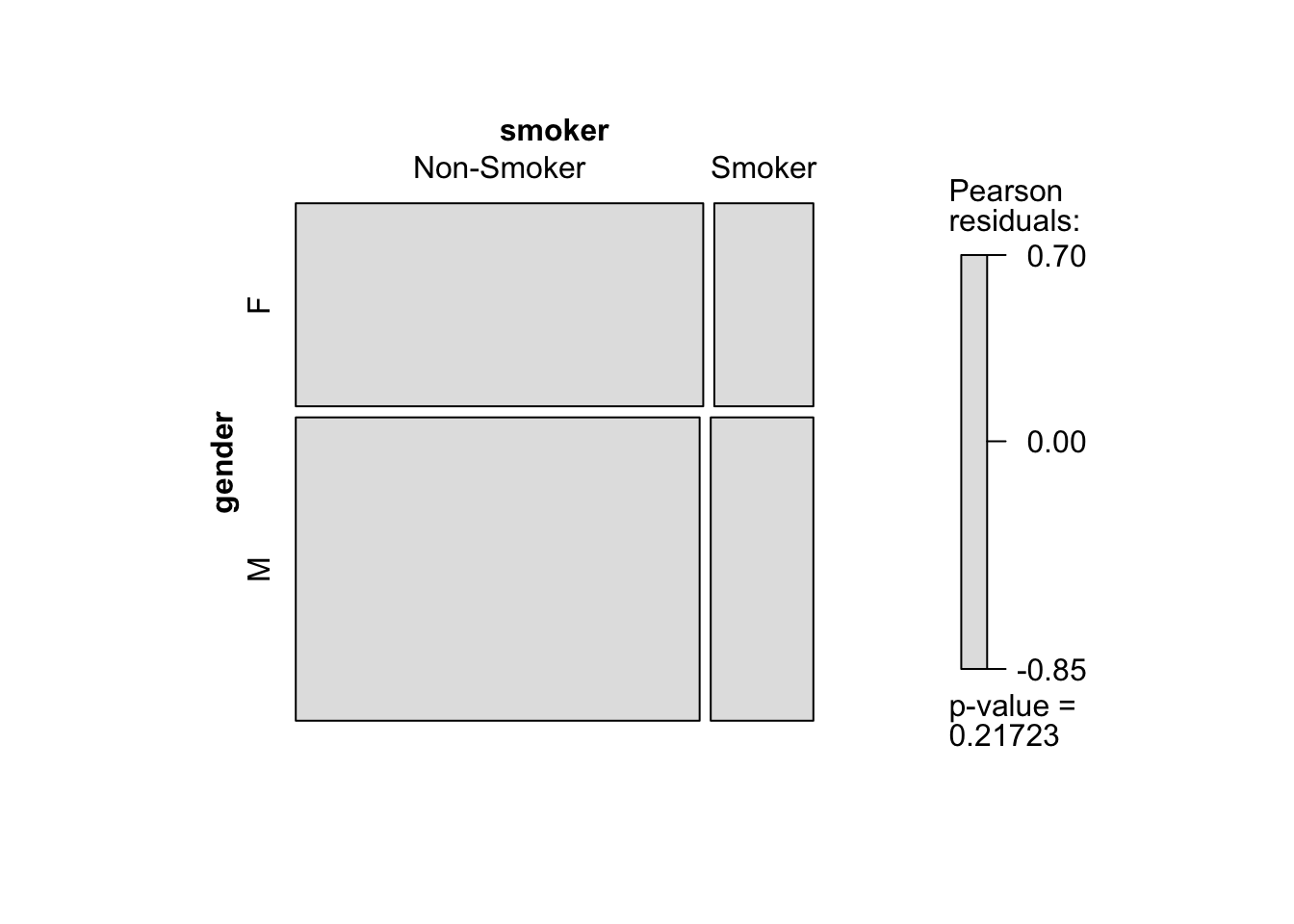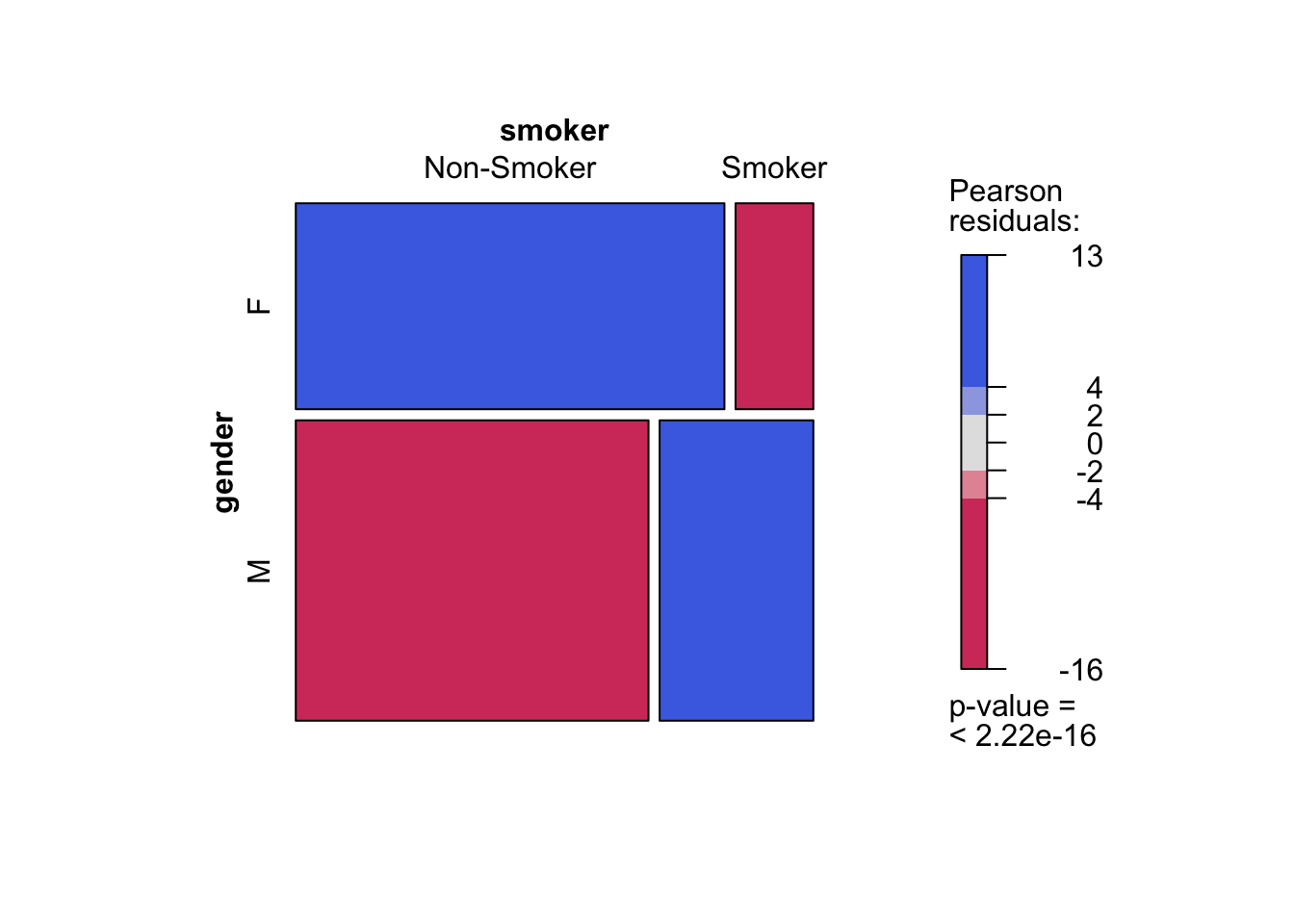## Setup

library(plyr) # Important that this one come first.
library(tidyverse)
## Loading tidyverse: ggplot2
## Loading tidyverse: dplyr
## Warning: package 'dplyr' was built under R version 3.4.2
## Conflicts with tidy packages ----------------------------------------------
## arrange():   dplyr, plyr
## compact():   purrr, plyr
## count():     dplyr, plyr
## failwith():  dplyr, plyr
## filter():    dplyr, stats
## id():        dplyr, plyr
## lag():       dplyr, stats
## mutate():    dplyr, plyr
## rename():    dplyr, plyr
## summarise(): dplyr, plyr
## summarize(): dplyr, plyr
library(vcd)
## Loading required package: grid
library(gmodels)

## Basic Concepts

The question we always ask with multiple categorical variables is similar to the question of correlation with quantitative variables. If we know the value of one variable, does that tell us anything about the likely value of another variable. If two quantitative variables are uncorrelated, the answer is no. In the case of two categorical variables, the amalogous concept is called independence. Two categorical variables are independent if the probability that one takes on a particular value does not depend on the value taken on by the other.

A population of people is 60% male and 40% female. 20% of the population smokes and 80% does not. Let’s simulate a population of 20,000 members with these characteristics.

# Create vectors to hold the characteristics.
gender = rep("",20000)
smoker = rep("",20000)

# Fill in the characteristics.
set.seed(1234)
for(i in 1:20000){
gender[i] = sample(c("M","F"), size = 1, c(.6,.4), replace = TRUE)
smoker[i] = sample(c("Smoker","Non-Smoker"), size = 1, c(.2,.8), replace = TRUE)
}
# Create a dataframe for the population
pI = data.frame(gender,smoker)

# Make sure our dataframe looks right.
str(pI)
## 'data.frame':    20000 obs. of  2 variables:
##  $gender: Factor w/ 2 levels "F","M": 2 1 1 2 1 1 2 2 2 2 ... ##$ smoker: Factor w/ 2 levels "Non-Smoker","Smoker": 1 1 1 1 1 1 2 2 1 1 ...

Let’s look at our population.

There are several ways to look at the relationship between gender and smoking. What we’re focusing on is the question of independence. Speaking of two events $$A$$ and $$B$$ in general, independence means that

$Prob(A & B) = Prob(A) * Prob(B)$.

Think about what this means for the approximate number of male smokers we should find in our population. The probability of being male is $$.6$$ and the probability of smoking is $$.2$$. So with independence, the probability of a randomly chosen person being male and a smoker is $$.6*.2=.12$$. Therefore with a population of $$20,000$$ we should find about $$20,000*.12=2,400$$ male smokers.

Let’s look at our population with the table() command from base R.

table(pI$gender,pI$smoker)
##
##     Non-Smoker Smoker
##   F       6451   1568
##   M       9553   2428

We actually get $$2,428$$ male smokers instead of $$2,400$$. How do we assess the challenge to independence represented by this difference? The standard way is to compute a chi-square statistic. Every cell in the table has a contribution to make. Consider the expected value, $$E$$ and the observed value, $$O$$. The chi-square contribution of an individual cell is given by

$\frac{(O-E)^2}{E}$. To get the chi-square statistic for the entire table, we add up the contributions from all of the cells. There is a function CrossTable() in the package gmodels, which does all of this and more. Let’s use this to look at our simulated population.

CrossTable(pI$gender,pI$smoker,
chisq = TRUE)
##
##
##    Cell Contents
## |-------------------------|
## |                       N |
## | Chi-square contribution |
## |           N / Row Total |
## |           N / Col Total |
## |         N / Table Total |
## |-------------------------|
##
##
## Total Observations in Table:  20000
##
##
##              | pI$smoker ## pI$gender | Non-Smoker |     Smoker |  Row Total |
## -------------|------------|------------|------------|
##            F |       6451 |       1568 |       8019 |
##              |      0.182 |      0.730 |            |
##              |      0.804 |      0.196 |      0.401 |
##              |      0.403 |      0.392 |            |
##              |      0.323 |      0.078 |            |
## -------------|------------|------------|------------|
##            M |       9553 |       2428 |      11981 |
##              |      0.122 |      0.489 |            |
##              |      0.797 |      0.203 |      0.599 |
##              |      0.597 |      0.608 |            |
##              |      0.478 |      0.121 |            |
## -------------|------------|------------|------------|
## Column Total |      16004 |       3996 |      20000 |
##              |      0.800 |      0.200 |            |
## -------------|------------|------------|------------|
##
##
## Statistics for All Table Factors
##
##
## Pearson's Chi-squared test
## ------------------------------------------------------------
## Chi^2 =  1.522574     d.f. =  1     p =  0.2172304
##
## Pearson's Chi-squared test with Yates' continuity correction
## ------------------------------------------------------------
## Chi^2 =  1.478375     d.f. =  1     p =  0.224029
##
## 

What’s the conclusion. The thing to look at is the “p-value.” With or without the Yates’ tweaking, its about 22%. This is the probability of observing a chi-square statistic this large if the hypothesis of independence is true. Since this is well above the standard 5% threshold, we have no reason to doubt independence.

We can use the mosaic() function from the vcd package to see this visually. Ina mosaic plot, the values in the cells are represented by the areas of the analogous parts of the plot. In this case, the deviation from independence is so small, that the plot is not very revealing.

mosaic(~ gender + smoker, data = pI,shade = TRUE)Now, let’s create a second population that demonstrates dependence.

# Create vectors to hold the characteristics.
gender = rep("",20000)
smoker = rep("",20000)

# Fill in the characteristics.
for(i in 1:20000){
gender[i] = sample(c("M","F"), size = 1, c(.6,.4), replace = TRUE)
if(gender[i] == "M"){
smoker[i] = sample(c("Smoker","Non-Smoker"), size = 1, c(.3,.7), replace = TRUE)
} else{
smoker[i] = sample(c("Smoker","Non-Smoker"), size = 1, c(.15,.85), replace = TRUE)
}

}
# Create a dataframe for the population
pD = data.frame(gender,smoker)

# Make sure our dataframe looks right.
str(pD)
## 'data.frame':    20000 obs. of  2 variables:
##  $gender: Factor w/ 2 levels "F","M": 2 2 2 1 2 2 1 1 1 2 ... ##$ smoker: Factor w/ 2 levels "Non-Smoker","Smoker": 1 1 2 1 1 1 1 1 1 2 ...

First, look at the CrossTable results.

CrossTable(pD$gender,pD$smoker,
chisq = TRUE)
##
##
##    Cell Contents
## |-------------------------|
## |                       N |
## | Chi-square contribution |
## |           N / Row Total |
## |           N / Col Total |
## |         N / Table Total |
## |-------------------------|
##
##
## Total Observations in Table:  20000
##
##
##              | pD$smoker ## pD$gender | Non-Smoker |     Smoker |  Row Total |
## -------------|------------|------------|------------|
##            F |       6885 |       1250 |       8135 |
##              |     84.968 |    265.271 |            |
##              |      0.846 |      0.154 |      0.407 |
##              |      0.455 |      0.258 |            |
##              |      0.344 |      0.062 |            |
## -------------|------------|------------|------------|
##            M |       8263 |       3602 |      11865 |
##              |     58.257 |    181.878 |            |
##              |      0.696 |      0.304 |      0.593 |
##              |      0.545 |      0.742 |            |
##              |      0.413 |      0.180 |            |
## -------------|------------|------------|------------|
## Column Total |      15148 |       4852 |      20000 |
##              |      0.757 |      0.243 |            |
## -------------|------------|------------|------------|
##
##
## Statistics for All Table Factors
##
##
## Pearson's Chi-squared test
## ------------------------------------------------------------
## Chi^2 =  590.3736     d.f. =  1     p =  2.078049e-130
##
## Pearson's Chi-squared test with Yates' continuity correction
## ------------------------------------------------------------
## Chi^2 =  589.5579     d.f. =  1     p =  3.126602e-130
##
## 

Note the differences in the chi-square contributions and the overall chi-square statistic. The p-value now indicates that the probability of seeing this result if the variables are independent is infinitesimal.

The mosaic plot is much more interesting.

mosaic(~ gender + smoker, data = pD,shade = TRUE)How do we read the table? Pearson residuals are derived from the chi-square contributions in such a way that they have standard normal(0,1) distributions for each cell under the hypothesis of independence. In this case, we see values 16 standard deviations from the mean in both directions. The blue values represent positive deviations, and the red values represent negative deviations. There are far more female non-smokers and male smokers that we would expect under independence. Similarly, there are far fewer female smokers and male non-smokers. The visualization is more informative than the table.